Function Repository Resource:

# EdgeColoring

Assign colors to edges of a graph so that no two adjacent edges have the same color

Contributed by: Wolfram Staff (original content by Sriram V. Pemmaraju and Steven S. Skiena)
 ResourceFunction["EdgeColoring"][g] gives an edge coloring of graph g.

## Details and Options

ResourceFunction["EdgeColoring"][g] separates edges of g into sublists such that no group contains adjacent edges—that is, edges that share a common vertex.
ResourceFunction["EdgeColoring"][g] uses the resource function VertexColoring to find a vertex coloring of the line graph of g.
ResourceFunction["EdgeColoring"] takes the Method option with the same possible values as in VertexColoring.
For simple graphs, the result of ResourceFunction["EdgeColoring"] can be used directly in HighlightGraph.
As in the resource function VertexColoring, ResourceFunction["EdgeColoring"] uses Brelaz's heuristic, which gives a good—but not necessarily minimal—coloring.
With Method"Optimum", ResourceFunction["EdgeColoring"] attempts to find a k-coloring with the smallest possible value of k (called the edge chromatic number, or chromatic index, of the graph).
ResourceFunction["EdgeColoring"] works with undirected graphs, directed graphs, multigraphs and mixed graphs, treating directed edges as undirected ones.

## Examples

### Basic Examples (4)

Get a sample graph:

 In:=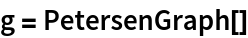Out=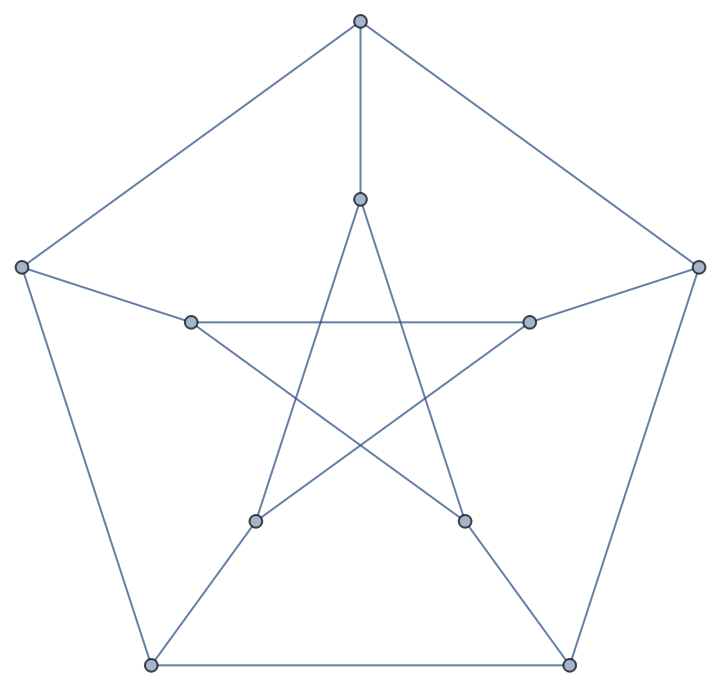Group edges of a graph so that no group contains adjacent edges:

 In:=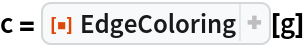Out=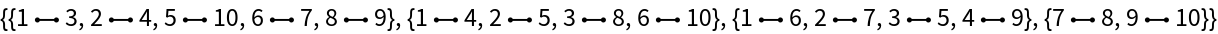Highlight edges in the groups:

 In:=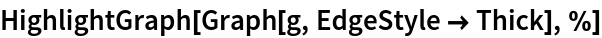Out=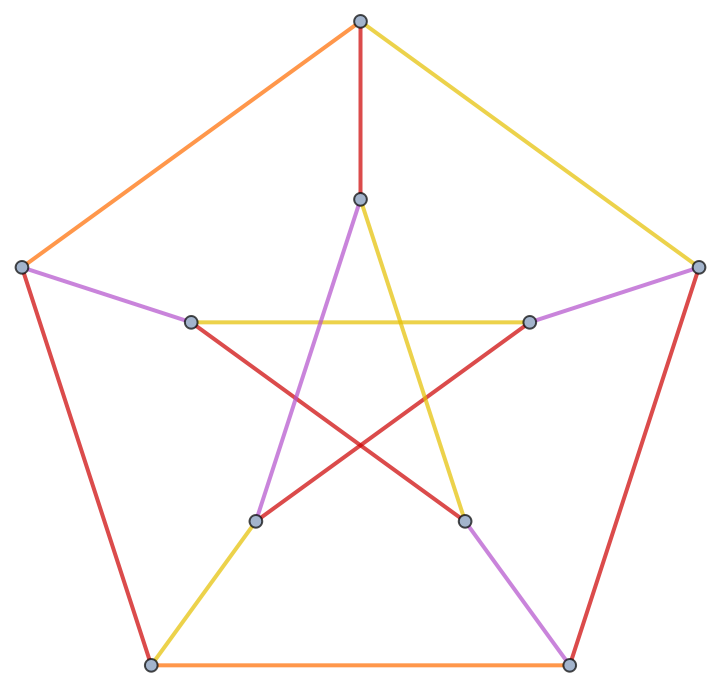Use the specified set of colors:

 In:=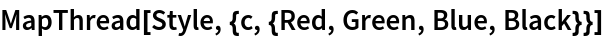Out=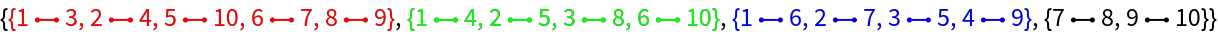In:=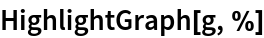Out=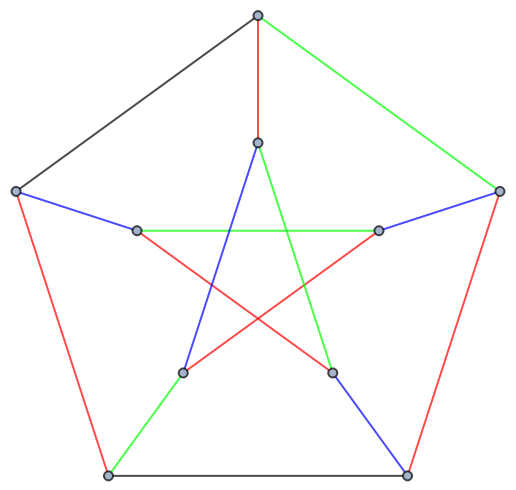### Scope (4)

EdgeColoring works with undirected graphs:

 In:=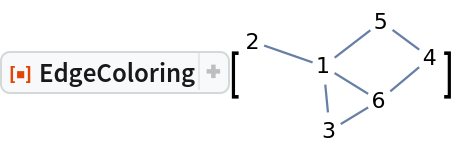Out=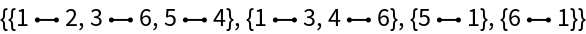Directed graphs:

 In:=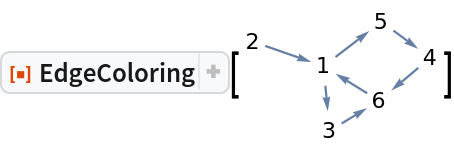Out=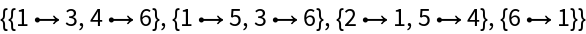Multigraphs:

 In:=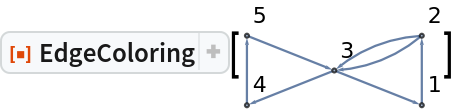Out=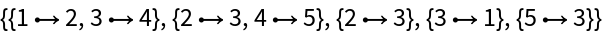Mixed graphs:

 In:=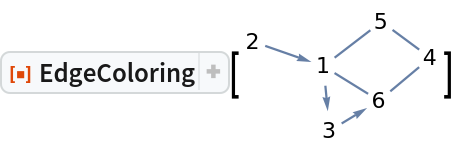Out=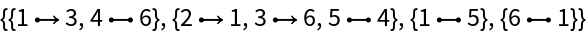### Options (4)

#### Method (4)

By default, EdgeColoring uses Brelaz's heuristic, which does not necessarily give the minimum coloring:

 In:=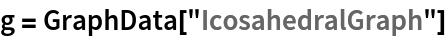Out=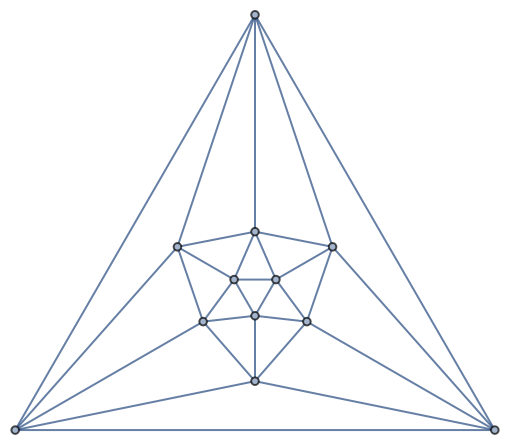In:=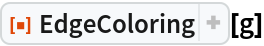Out=In this case, a 6-coloring is produced:

 In:=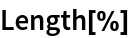Out=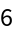The minimum coloring should use only five colors, as given by the chromatic number of the graph:

 In:=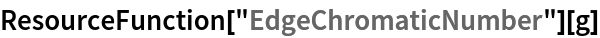Out=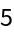Obtain a minimum coloring using Method"Optimum":

 In:=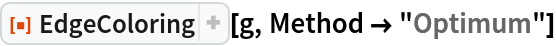Out=In:=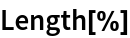Out=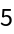In:=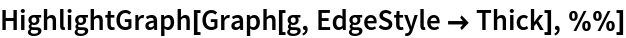Out=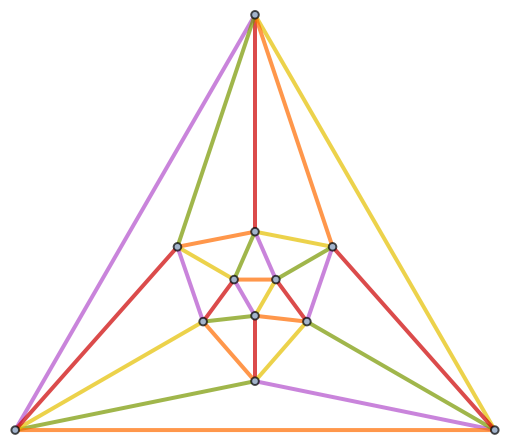### Properties and Relations (2)

EdgeColoring returns a proper edge coloring–that is, a coloring in which no two adjacent edges share the same color:

 In:=In:=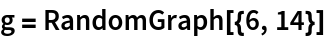Out=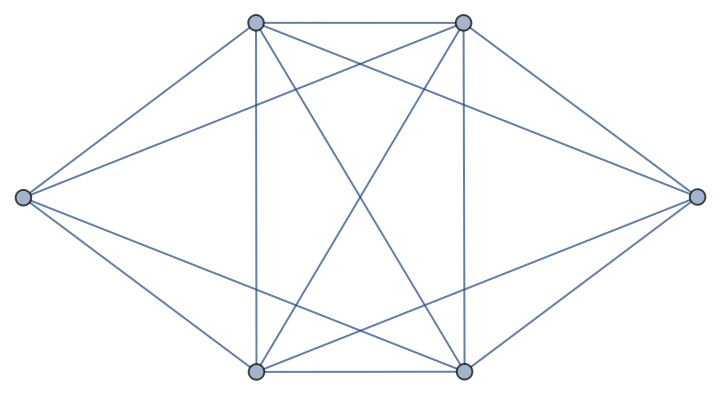In:=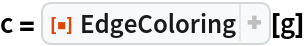Out=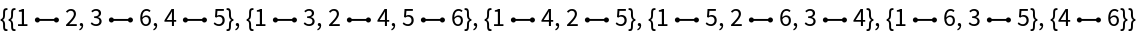In:=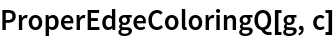Out=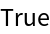Verify by inspection:

 In:=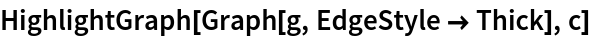Out=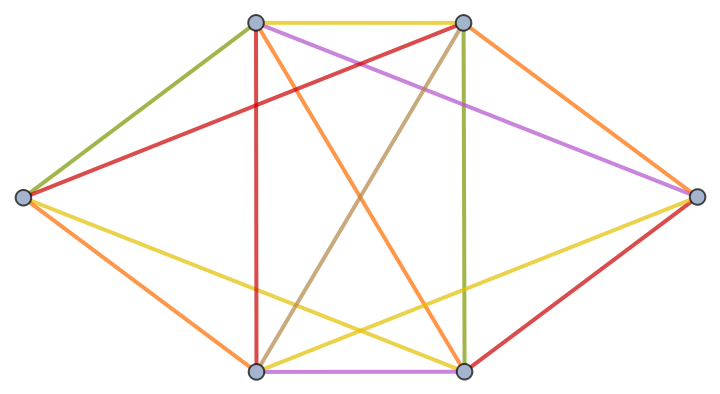Use EdgeTaggedGraph to verify an edge coloring of a multigraph by inspection:

 In:=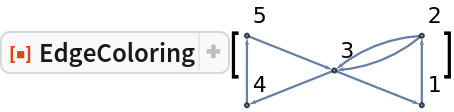Out=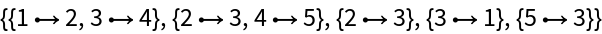In:=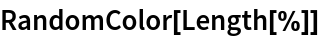Out=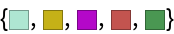In:=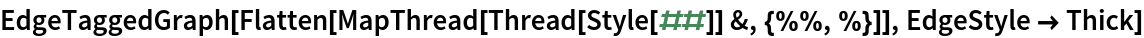Out=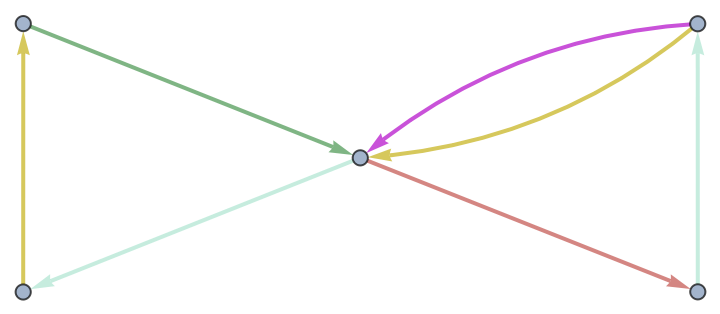## Version History

• 1.0.0 – 22 October 2020